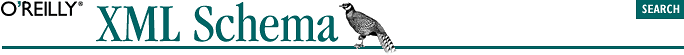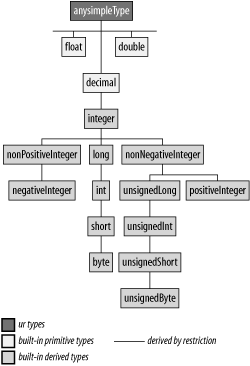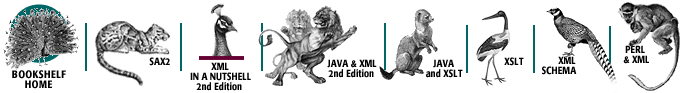home | O'Reilly's CD bookshelfs | FreeBSD | Linux | Cisco | Cisco Exam## 4.4. Numeric Datatypes

The numeric datatypes are built on top of four primitive datatypes: xs:decimal for all the decimal types (including the integer datatypes, considered decimals without a fractional part), xs:double and xs:float for single and double precision floats, and xs:boolean for Booleans. Whitespaces are collapsed for all these datatypes.

The datatypes covered in this section are shown in Figure 4-3.### 4.4.1. Decimal Types

All decimal types are derived from the xs:decimal primary type and constitute a set of predefined types that address the most common usages.

xs:decimal
This datatype represents the decimal numbers. The number of digits can be arbitrarily long (the datatype doesn't impose any restriction), but obviously, since a XML document has an arbitrary but finite length, the number of digits of the lexical representation of a xs:decimal value needs to be finite. Although the number of digits is not limited, we will see in the next chapter how the author of a schema can derive user-defined datatypes with a limited number of digits if needed.

Leading and trailing zeros are not significant and may be trimmed. The decimal separator is always a dot ("."); a leading sign ("+" or "-") may be specified and any characters other than the 10 digits (including whitespaces) are forbidden. Scientific notation ("E+2") is also forbidden and has been reserved to the float datatypes only.

Valid values for xs:decimal include:

123.456

+1234.456

-1234.456

-.456

-456

The following values are invalid:

1 234.456 (spaces are forbidden)

1234.456E+2 (scientific notation ("E+2") is forbidden)

+ 1234.456 (spaces are forbidden)

+1,234.456 (delimiters between thousands are forbidden)

xs:integer is the only datatype directly derived from xs:decimal.

xs:integer
This integer datatype is a subset of xs:decimal, representing numbers which don't have any fractional digits in its lexical or value spaces. The characters that are accepted are reduced to 10 digits and an optional leading sign. Like its base datatype, xs:integer doesn't impose any limitation on the number of digits, and leading zeros are not significant.

Valid values for xs:integer include:

123456

+00000012

-1

-456

The following values are invalid:

1 234 (spaces are forbidden)

1. (the decimal separator is forbidden)

+1,234 (delimiters between thousands are forbidden).

xs:integer has given birth to three derived datatypes: xs:nonPositiveInteger and xs:nonNegativeInteger (which have still an unlimited length) and xs:long (to fit in a 64-bit word).

xs:nonPositiveInteger and xs:negativeInteger
The W3C XML Schema Working Group thought that it would be more clear that the value "0" was included if they used litotes as names, and used xs:nonPositiveInteger if the integers are negative or null. xs:negativeInteger is derived from xs:nonPositiveInteger to represent the integers that are strictly negative. These two datatypes allow integers of arbitrary length.

xs:nonNegativeInteger and xs:positiveInteger
Similarly, xs:nonNegativeInteger is the integers that are positive or equal to zero and xs:positiveInteger is derived from this type. The "unsigned" family branch (xs:unsignedLong, xs:unsignedInt, xs:unsignedShort, and xs:unsignedByte) is also derived from xs:nonNegativeInteger.

xs:long, xs:int, xs:short, and xs:byte.
The datatypes we have seen up to now have an unconstrained length. This approach isn't very microprocessor-friendly. This subfamily represents signed integers that can fit into 8, 16, 32, and 64-bit words. xs:long is defined as all of the integers between -9223372036854775808 and 9223372036854775807, i.e., the values that can be stored in a 64-bit word. The same process is applied again to derive xs:int with a range between -2147483648 and 2147483647 (32 bits), to derive xs:short with a range between -32768 and 32767 (16 bits), and to derive xs:byte with a range between -128 and 127 (8 bits).

xs:unsignedLong, xs:unsignedInt, xs:unsignedShort, and xs:unsignedByte.
The last of the predefined integer datatypes is the subfamily of unsigned (i.e., positive) integers that can fit into 8, 16, 32, and 64-bit words. xs:unsignedLong is defined as the integers in a range between 0 and 18446744073709551615, i.e., the values that can be stored in a 64-bit word. The same process is applied again to derive xs:unsignedInt with a range between 0 and 4294967295 (32 bits), to derive xs:unsignedShort with a range between 0 and 65535 (16 bits), and to derive xs:unsignedByte with a range between 0 and 255 (8 bits).

### 4.4.2. Float Datatypes

xs:float and xs:double
xs:float and xs:double are both primitive datatypes and represent IEEE simple (32 bits) and double (64 bits) precision floating-point types. These store the values in the form of mantissa and an exponent of a power of 2 (m x 2^e), allowing a large scale of numbers in a storage that has a fixed length. Fortunately, the lexical space doesn't require that we use powers of 2 (in fact, it doesn't accept powers of 2), but instead lets us use a traditional scientific notation with integer powers of 10. Since the value spaces (powers of 2) don't exactly match the values from the lexical space (powers of 10), the recommendation specifies that the closest value is taken. The consequence of this approximate matching is that float datatypes are the domain of approximation; most of the float values can't be considered exact, and are approximate.

These datatypes accept several "special" values: positive zero (0), negative zero (-0) (which is greater than positive 0 but less than any negative value), infinity (INF) (which is greater than any value), negative infinity (-INF) (which is less than any float, and "not a number" (NaN).

Valid values for xs:float and xs:double include:

123.456

+1234.456

-1.2344e56

-.45E-6

INF

-INF

NaN

The following values are invalid:

1234.4E 56 (spaces are forbidden)

1E+2.5 (the power of 10 must be an integer)

+INF (positive infinity doesn't expect a sign)

NAN (capitalization matters in special values)